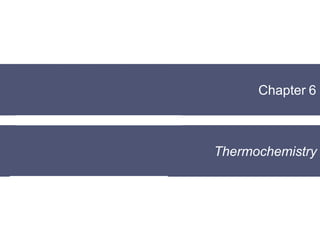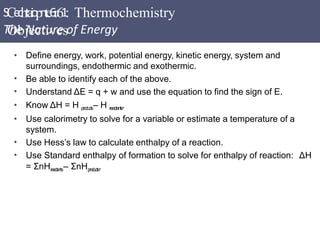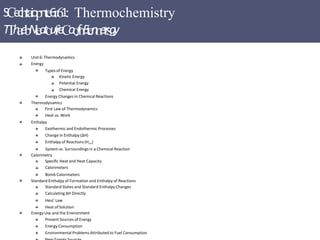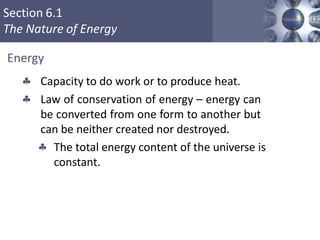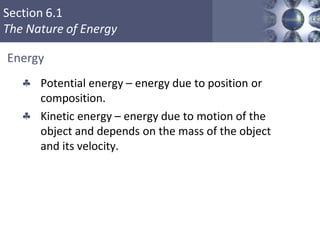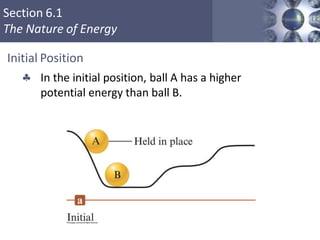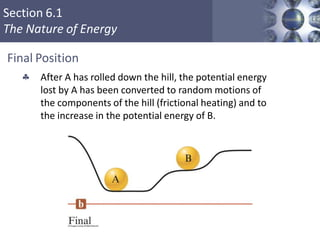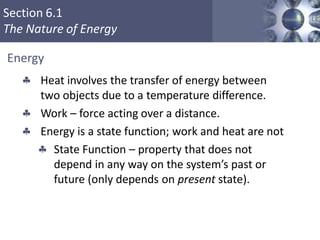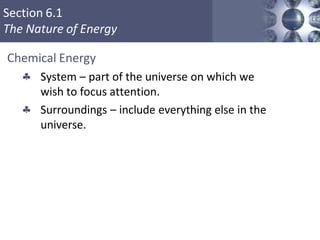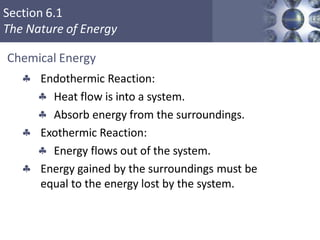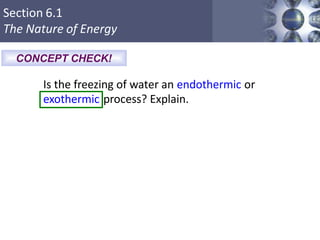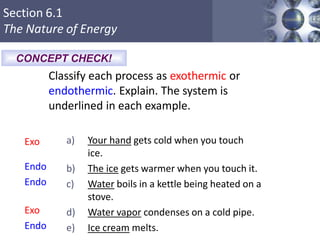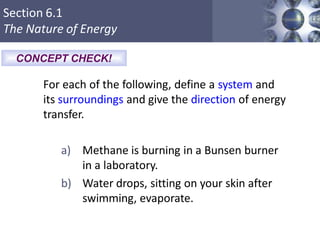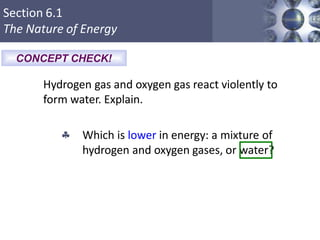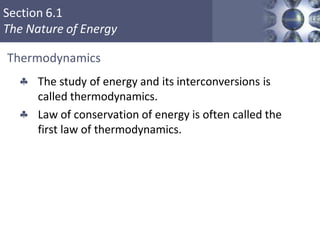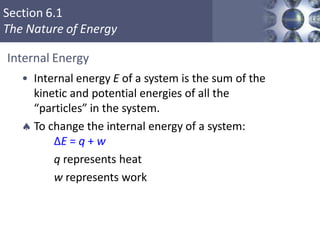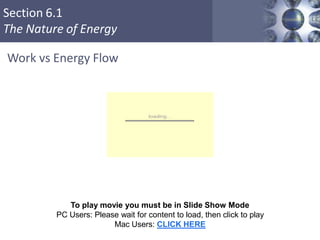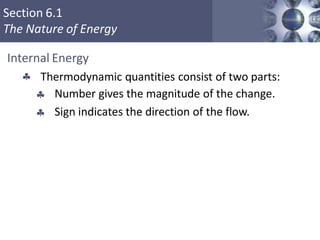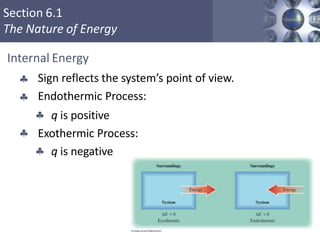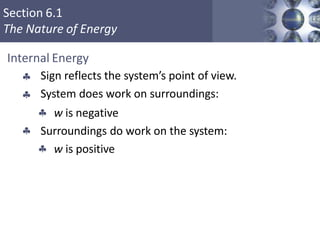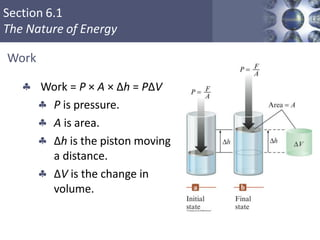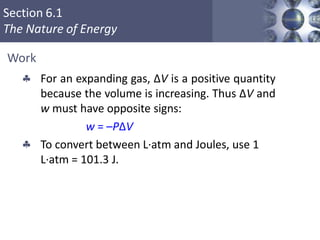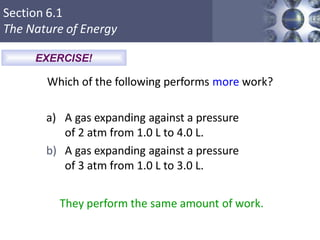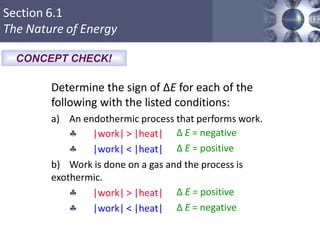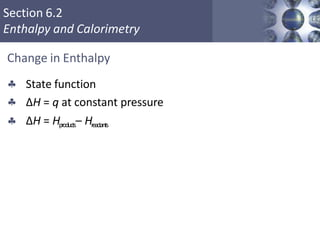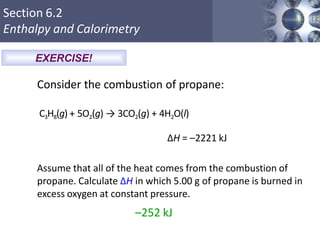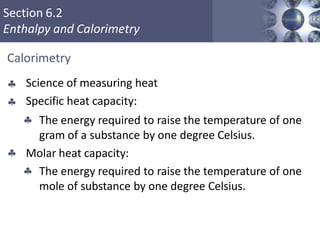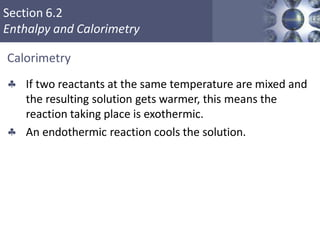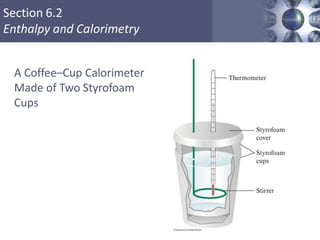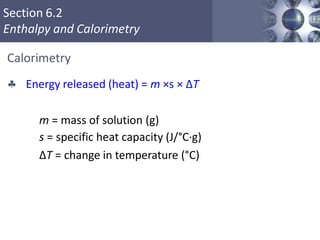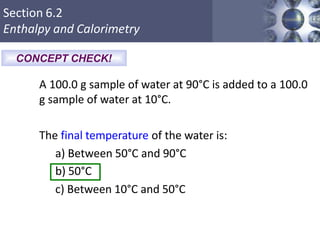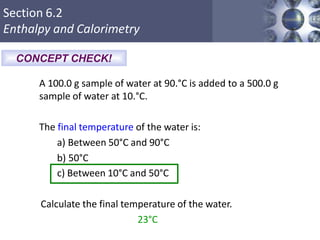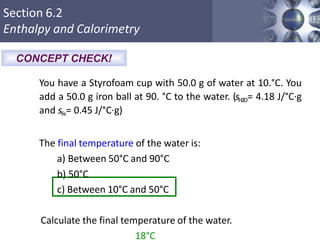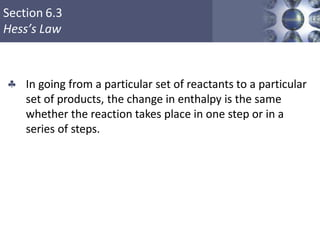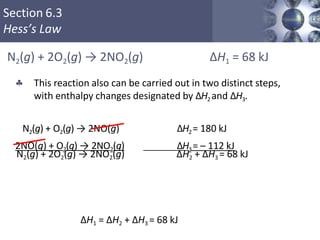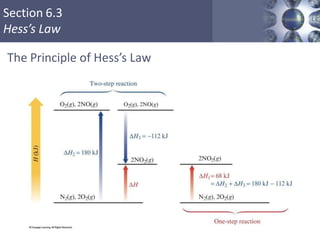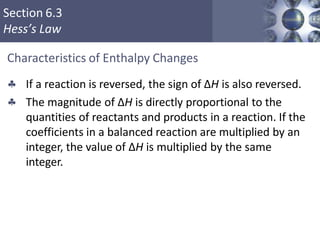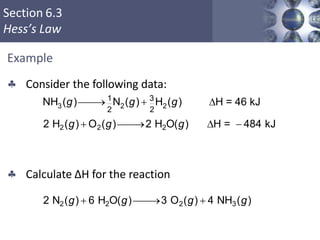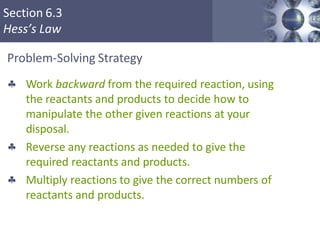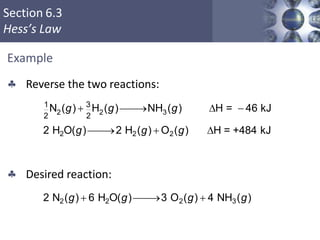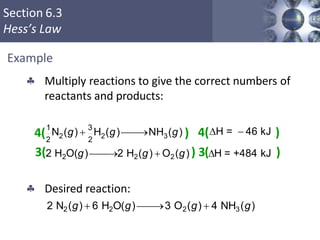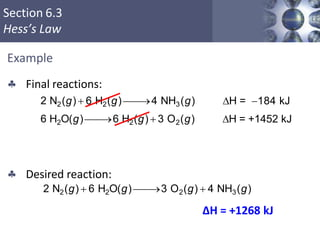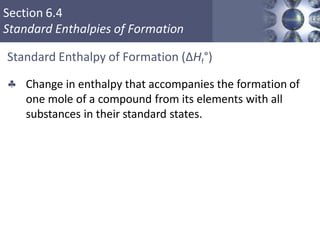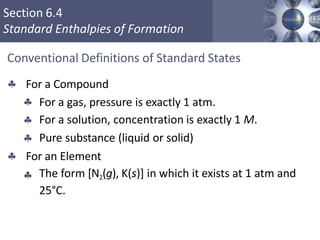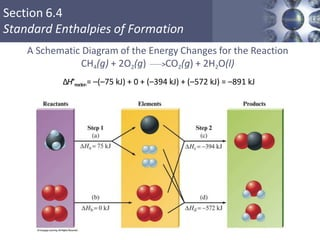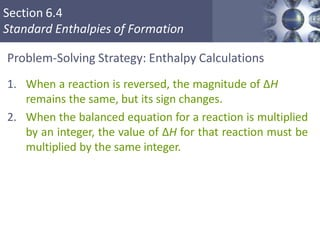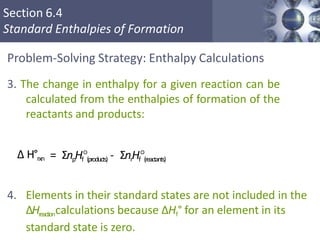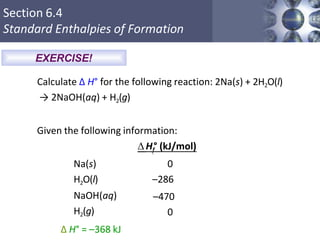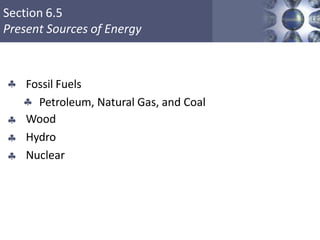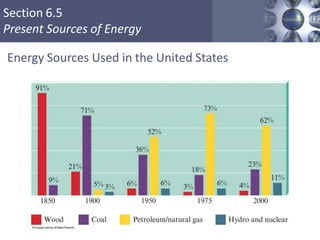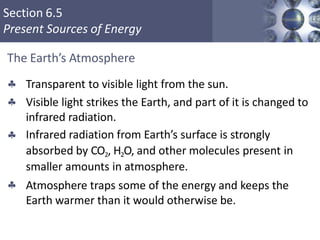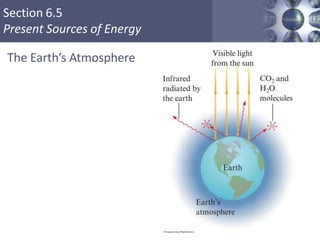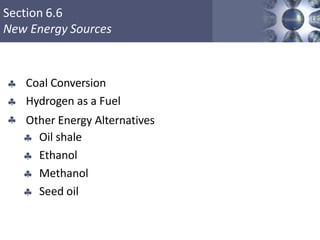1 of 55

### thermochemistry.pptx

1. Chapter 6 Thermochemistry
2. SCechtaiopnte6r.61: Thermochemistry TO he bjN ea ct ti u v re esof Energy • • • • • • • Define energy, work, potential energy, kinetic energy, system and surroundings, endothermic and exothermic. Be able to identify each of the above. Understand ΔE = q + w and use the equation to find the sign of E. Know ΔH = H p r o d u c t s– H reactants. Use calorimetry to solve for a variable or estimate a temperature of a system. Use Hess’s law to calculate enthalpy of a reaction. Use Standard enthalpy of formation to solve for enthalpy of reaction: ΔH = ΣnHre a cta n ts– ΣnHp ro d u c ts.
3. SCechtaiopnte6r.61: Thermochemistry T Th ae bN lea t ou fr e Co of nE ten ne tr sg y   Unit 6: Thermodynamics Energy  Types of Energy    Kinetic Energy Potential Energy Chemical Energy  Energy Changes in Chemical Reactions  Thermodynamics   First Law of Thermodynamics Heat vs. Work  Enthalpy  Exothermic and Endothermic Processes  Change in Enthalpy (ΔH)  Enthalpy of Reactions (Hrxn)  System vs. Surroundings in a Chemical Reaction  Calorimetry    Specific Heat and Heat Capacity Calorimeters Bomb Calorimeters  Standard Enthalpy of Formation and Enthalpy of Reactions     Standard States and Standard Enthalpy Changes Calculating ΔH Directly Hess’ Law Heat of Solution  Energy Use and the Environment  Present Sources of Energy  Energy Consumption  Environmental Problems Attributed to Fuel Consumption
4. Section 6.1 The Nature of Energy   Capacity to do work or to produce heat. Law of conservation of energy – energy can be converted from one form to another but can be neither created nor destroyed.  The total energy content of the universe is constant. Energy
5. Section 6.1 The Nature of Energy  Potential energy – energy due to position or composition. Kinetic energy – energy due to motion of the object and depends on the mass of the object and its velocity.  Energy
6. Section 6.1 The Nature of Energy  In the initial position, ball A has a higher potential energy than ball B. Initial Position
7. Section 6.1 The Nature of Energy  After A has rolled down the hill, the potential energy lost by A has been converted to random motions of the components of the hill (frictional heating) and to the increase in the potential energy of B. Final Position
8. Section 6.1 The Nature of Energy  Heat involves the transfer of energy between two objects due to a temperature difference. Work – force acting over a distance. Energy is a state function; work and heat are not    State Function – property that does not depend in any way on the system’s past or future (only depends on present state). Energy
9. Section 6.1 The Nature of Energy  System – part of the universe on which we wish to focus attention. Surroundings – include everything else in the universe.  Chemical Energy
10. Section 6.1 The Nature of Energy  Endothermic Reaction:   Heat flow is into a system. Absorb energy from the surroundings.  Exothermic Reaction:  Energy flows out of the system.  Energy gained by the surroundings must be equal to the energy lost by the system. Chemical Energy
11. Section 6.1 The Nature of Energy exothermic process? Explain. CONCEPT CHECK! Is the freezing of water an endothermic or
12. Section 6.1 The Nature of Energy a) Your hand gets cold when you touch ice. b) The ice gets warmer when you touch it. c) Water boils in a kettle being heated on a stove. d) Water vapor condenses on a cold pipe. e) Ice cream melts. Exo Endo Endo Exo Endo CONCEPT CHECK! Classify each process as exothermic or endothermic. Explain. The system is underlined in each example.
13. Section 6.1 The Nature of Energy CONCEPT CHECK! For each of the following, define a system and its surroundings and give the direction of energy transfer. a) Methane is burning in a Bunsen burner in a laboratory. b) Water drops, sitting on your skin after swimming, evaporate.
14. Section 6.1 The Nature of Energy  Which is lower in energy: a mixture of hydrogen and oxygen gases, or water? CONCEPT CHECK! Hydrogen gas and oxygen gas react violently to form water. Explain.
15. Section 6.1 The Nature of Energy Thermodynamics  The study of energy and its interconversions is called thermodynamics. Law of conservation of energy is often called the first law of thermodynamics. 
16. Section 6.1 The Nature of Energy Internal Energy • Internal energy E of a system is the sum of the kinetic and potential energies of all the “particles” in the system.  To change the internal energy of a system: ΔE = q + w q represents heat w represents work
17. Section 6.1 The Nature of Energy Work vs Energy Flow To play movie you must be in Slide Show Mode PC Users: Please wait for content to load, then click to play Mac Users: CLICK HERE
18. Section 6.1 The Nature of Energy  Thermodynamic quantities consist of two parts:   Number gives the magnitude of the change. Sign indicates the direction of the flow. Internal Energy
19. Section 6.1 The Nature of Energy Internal Energy   Sign reflects the system’s point of view. Endothermic Process:  q is positive  Exothermic Process:  q is negative
20. Section 6.1 The Nature of Energy   Sign reflects the system’s point of view. System does work on surroundings:  w is negative  Surroundings do work on the system:  w is positive Internal Energy
21. Section 6.1 The Nature of Energy  Work = P × A × Δh = PΔV    P is pressure. A is area. Δh is the piston moving a distance. ΔV is the change in volume.  Work
22. Section 6.1 The Nature of Energy Work  For an expanding gas, ΔV is a positive quantity because the volume is increasing. Thus ΔV and w must have opposite signs: w = –PΔV To convert between L·atm and Joules, use 1 L·atm = 101.3 J. 
23. Section 6.1 The Nature of Energy EXERCISE! Which of the following performs more work? a) A gas expanding against a pressure of 2 atm from 1.0 L to 4.0 L. b) A gas expanding against a pressure of 3 atm from 1.0 L to 3.0 L. They perform the same amount of work.
24. Section 6.1 The Nature of Energy   |work| > |heat| |work| < |heat| b) Work is done on a gas and the process is exothermic.   |work| > |heat| |work| < |heat| Δ E = negative Δ E = positive Δ E = positive Δ E = negative CONCEPT CHECK! Determine the sign of ΔE for each of the following with the listed conditions: a) An endothermic process that performs work.
25. Section 6.2 Enthalpy and Calorimetry Change in Enthalpy    State function ΔH = q at constant pressure ΔH = Hproducts– Hreactants
26. Section 6.2 Enthalpy and Calorimetry EXERCISE! Consider the combustion of propane: C3H8(g) + 5O2(g) → 3CO2(g) + 4H2O(l) ΔH = –2221 kJ Assume that all of the heat comes from the combustion of propane. Calculate ΔH in which 5.00 g of propane is burned in excess oxygen at constant pressure. –252 kJ
27. Section 6.2 Enthalpy and Calorimetry Calorimetry   Science of measuring heat Specific heat capacity:  The energy required to raise the temperature of one gram of a substance by one degree Celsius.  Molar heat capacity:  The energy required to raise the temperature of one mole of substance by one degree Celsius.
28. Section 6.2 Enthalpy and Calorimetry Calorimetry  If two reactants at the same temperature are mixed and the resulting solution gets warmer, this means the reaction taking place is exothermic. An endothermic reaction cools the solution. 
29. Section 6.2 Enthalpy and Calorimetry A Coffee–Cup Calorimeter Made of Two Styrofoam Cups
30. Section 6.2 Enthalpy and Calorimetry Calorimetry  Energy released (heat) = m ×s × ΔT m = mass of solution (g) s = specific heat capacity (J/°C·g) ΔT = change in temperature (°C)
31. Section 6.2 Enthalpy and Calorimetry b) 50°C c) Between 10°C and 50°C CONCEPT CHECK! A 100.0 g sample of water at 90°C is added to a 100.0 g sample of water at 10°C. The final temperature of the water is: a) Between 50°C and 90°C
32. Section 6.2 Enthalpy and Calorimetry c) Between 10°C and 50°C Calculate the final temperature of the water. 23°C CONCEPT CHECK! A 100.0 g sample of water at 90.°C is added to a 500.0 g sample of water at 10.°C. The final temperature of the water is: a) Between 50°C and 90°C b) 50°C
33. Section 6.2 Enthalpy and Calorimetry c) Between 10°C and 50°C Calculate the final temperature of the water. 18°C CONCEPT CHECK! You have a Styrofoam cup with 50.0 g of water at 10.°C. You add a 50.0 g iron ball at 90. °C to the water. (sH2O= 4.18 J/°C·g and sFe= 0.45 J/°C·g) The final temperature of the water is: a) Between 50°C and 90°C b) 50°C
34. Section 6.3 Hess’s Law  In going from a particular set of reactants to a particular set of products, the change in enthalpy is the same whether the reaction takes place in one step or in a series of steps.
35. Section 6.3 Hess’s Law  This reaction also can be carried out in two distinct steps, with enthalpy changes designated by ΔH2 and ΔH3. N2(g) + O2(g) → 2NO(g) ΔH2= 180 kJ 2NO(g) + O2(g) → 2NO2(g) ΔH3= – 112 kJ N2(g) + 2O2(g) → 2NO2(g) ΔH1 = 68 kJ N2(g) + 2O2(g) → 2NO2(g) ΔH2 + ΔH3 = 68 kJ ΔH1 = ΔH2 + ΔH3 = 68 kJ
36. Section 6.3 Hess’s Law The Principle of Hess’s Law
37. Section 6.3 Hess’s Law To play movie you must be in Slide Show Mode PC Users: Please wait for content to load, then click to play Mac Users: CLICK HERE
38. Section 6.3 Hess’s Law Characteristics of Enthalpy Changes   If a reaction is reversed, the sign of ΔH is also reversed. The magnitude of ΔH is directly proportional to the quantities of reactants and products in a reaction. If the coefficients in a balanced reaction are multiplied by an integer, the value of ΔH is multiplied by the same integer.
39. Section 6.3 Hess’s Law Example  Consider the following data:  Calculate ΔH for the reaction 3 2 2 2 H2(g)  O2(g)  2 H2O(g) 2 2 NH (g)   1 N (g)  3 H (g) H = 46 kJ H =  484 kJ 2 N2(g)  6 H2O(g)  3 O2(g)  4 NH3(g)
40. Section 6.3 Hess’s Law Problem-Solving Strategy  Work backward from the required reaction, using the reactants and products to decide how to manipulate the other given reactions at your disposal. Reverse any reactions as needed to give the required reactants and products. Multiply reactions to give the correct numbers of reactants and products.  
41. Section 6.3 Hess’s Law Example  Reverse the two reactions:  Desired reaction: 2 N2(g)  6 H2O(g)  3 O2(g)  4 NH3(g) 2 2 3 2 H2O(g)  2 H2(g)  O2(g) 2 2 1 N (g)  3 H (g)  NH (g) H =  46 kJ H = +484 kJ
42. Section 6.3 Hess’s Law Example  Multiply reactions to give the correct numbers of reactants and products: 4( ) 4(H =  46 kJ )  Desired reaction: 2 N2(g)  6 H2O(g)  3 O2(g)  4 NH3(g) 2 2 3 1 3 2 2 N (g)  H (g)  NH (g) 3(2 H2O(g) 2 H2(g)  O2(g) ) 3(H = +484 kJ )
43. Section 6.3 Hess’s Law Example  Final reactions: 2 N2(g)  6 H2(g)  4 NH3(g) 6 H2O(g)  6 H2(g)  3 O2(g)  Desired reaction: 2 N2(g)  6 H2O(g)  3 O2(g)  4 NH3(g) ΔH = +1268 kJ H = 184 kJ H = +1452 kJ
44. Section 6.4 Standard Enthalpies of Formation Standard Enthalpy of Formation (ΔHf°)  Change in enthalpy that accompanies the formation of one mole of a compound from its elements with all substances in their standard states.
45. Section 6.4 Standard Enthalpies of Formation Conventional Definitions of Standard States  For a Compound    For a gas, pressure is exactly 1 atm. For a solution, concentration is exactly 1 M. Pure substance (liquid or solid)  For an Element  The form [N2(g), K(s)] in which it exists at 1 atm and 25°C.
46. Section 6.4 Standard Enthalpies of Formation A Schematic Diagram of the Energy Changes for the Reaction CH4(g) + 2O2(g) CO2(g) + 2H2O(l) ΔH°reaction= –(–75 kJ) + 0 + (–394 kJ) + (–572 kJ) = –891 kJ
47. Section 6.4 Standard Enthalpies of Formation Problem-Solving Strategy: Enthalpy Calculations 1. When a reaction is reversed, the magnitude of ΔH remains the same, but its sign changes. 2. When the balanced equation for a reaction is multiplied by an integer, the value of ΔH for that reaction must be multiplied by the same integer.
48. Section 6.4 Standard Enthalpies of Formation Problem-Solving Strategy: Enthalpy Calculations 3. The change in enthalpy for a given reaction can be calculated from the enthalpies of formation of the reactants and products: Δ H°rxn = ΣnpHf(products) - ΣnrHf(reactants) 4. Elements in their standard states are not included in the ΔHreactioncalculations because ΔHf° for an element in its standard state is zero.
49. Section 6.4 Standard Enthalpies of Formation Na(s) H2O(l) NaOH(aq) H2(g) Δ H° = –368 kJ 0 –286 –470 0 EXERCISE! Calculate Δ H° for the following reaction: 2Na(s) + 2H2O(l) → 2NaOH(aq) + H2(g) Given the following information:  Hf° (kJ/mol)
50. Section 6.5 Present Sources of Energy  Fossil Fuels  Petroleum, Natural Gas, and Coal    Wood Hydro Nuclear
51. Section 6.5 Present Sources of Energy Energy Sources Used in the United States
52. Section 6.5 Present Sources of Energy The Earth’s Atmosphere   Transparent to visible light from the sun. Visible light strikes the Earth, and part of it is changed to infrared radiation. Infrared radiation from Earth’s surface is strongly absorbed by CO2, H2O, and other molecules present in smaller amounts in atmosphere. Atmosphere traps some of the energy and keeps the Earth warmer than it would otherwise be.  
53. Section 6.5 Present Sources of Energy The Earth’s Atmosphere
54. Section 6.6 New Energy Sources    Coal Conversion Hydrogen as a Fuel Other Energy Alternatives     Oil shale Ethanol Methanol Seed oil
55. Section 6.6 New Energy Sources Chapter 6 Activity